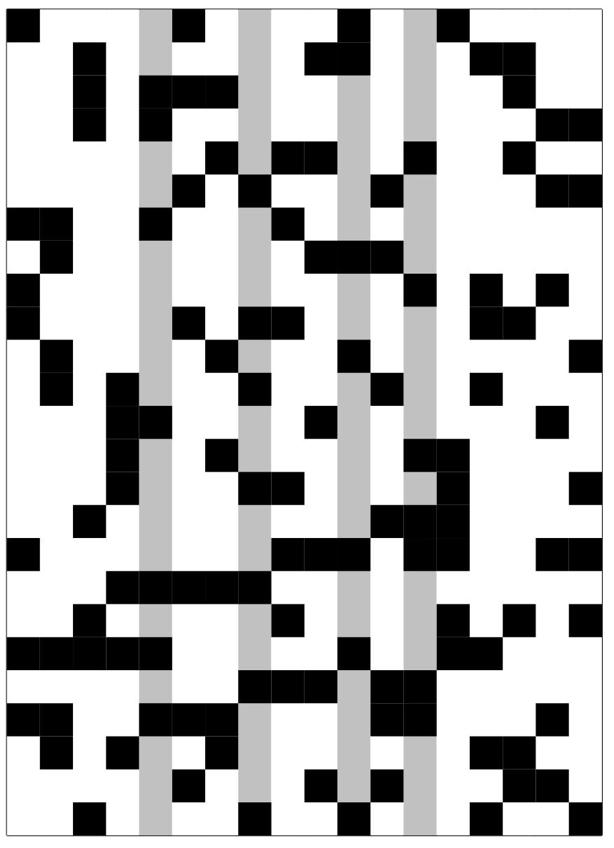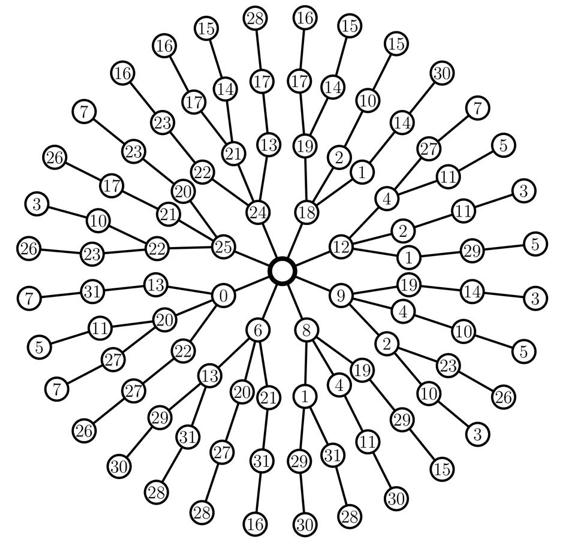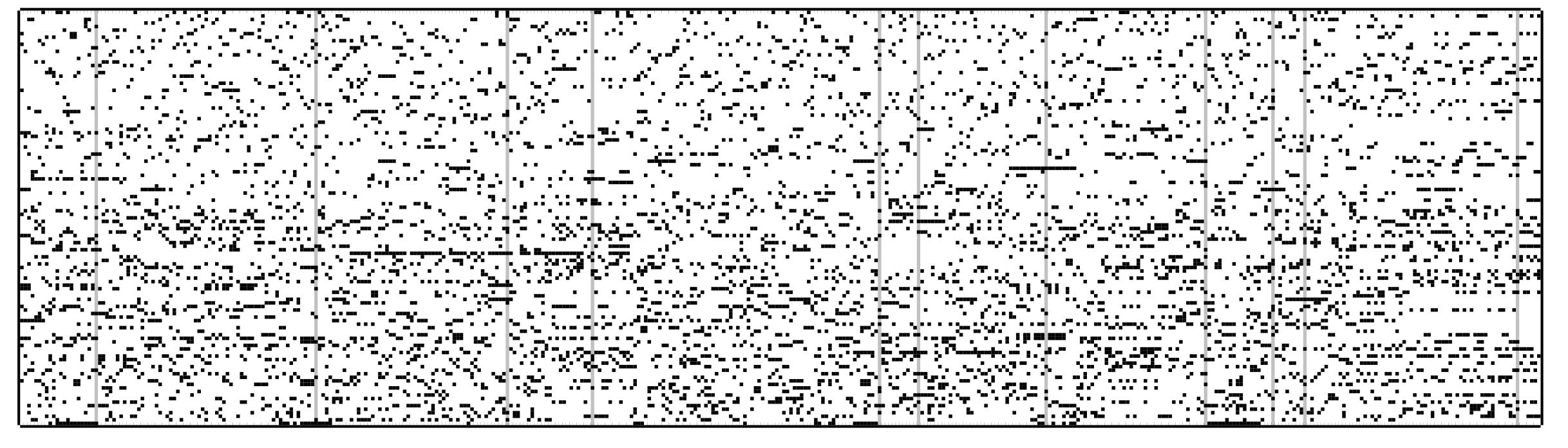## Anton Betten: Research

I have been working on the problem of classifying combinatorial objects. Often, the search for combinatorial objects can be expressed in terms of NP-complete problems from Computer Science, like the
exact cover problem
or the
clique problem
or slight modifications of these problems.

Before we proceed, let us hold on for a while and think what it means to work on NP-complete problems. It is highly unlikely that someone will come up with polynomial time algorithms anytime soon. Does that mean we should not solve on problems like these?
Peter Cameron has put it this way:
That a problem is hard does not mean we should not solve it.
Many interesting mathematical problems can be reduced to NP-complete problems. I wish to illustrate this reduction with some examples from my work. Basically, we take a classification problem in combinatorics and transform it into a set of instances of exact cover or rainbow clique. These instances are solved and the solutions are obtained. Then we do some post-processing in order to achieve the classification.

The following questions arise: How do we tranform a mathematical problem into instances of exact cover or clique finding or versions of it? What algorithms do we use to solve these problems? Where do we run the software? Do we have the computational power to run sufficiently large and interesting cases? What kind of post-processing is needed once we have the solutions? All these are questions that need to be worked out.

For now, let us not get lost in details. Let us look at the kind of computer science primitives that we need.

## Exact Cover

Let us look at the exact cover problem. The problem can be phrased as solving a system
 A·x = 1
where A is a matrix with coefficients in 0 and 1 (known), 1 is the vector of all ones, and x is a vector of 0 and 1 (unknown). Each row of A can be thought of as a condition of covering something exactly once. The columns of A can be thought of as the pieces with which we cover. The ones in the solution vector x tell us which pieces we choose.

The graphic below tries to illustrate this method. It is from a problem of classifying a type of structure known as a dual hyperoval. The rows in the matrix represent the equations, and a coefficient of one is indicated by a black box. The nature of this particular system is that we want to choose 4 variables one and all others zero. The condition is that the first few equations have right hand side one. The equations at the bottom have right hand side either zero or two. The 4 selected coefficients correspond to the 4 columns that are gray. A computer program can find all ways in which the columns can be selected according to the conditions described by the matrix and the right hand side. Of course, this system is for illustration purposes only. In research problems, the systems that we solve are much larger.Here is a system with 32 columns. The computer found 4 columns (indicated in gray) which satisfy the conditions. The program performs a backtrack search to examine all possibilities. This particular system requires about 97 search steps. Again, this selected system is very small.Here is the search tree. The root of the tree is at the center of the drawing. The search proceeds outwards from the root. The solution that is indicated above corresponds to the branch 0−13−31−7 in the search tree. Observe how the search algorithm picks the columns in a seemlingly random order. However, the order is not random. It is determined by the algorithm and it is chosen so that the search tree is kept small. Also, observe that the subtrees of the root node are all identical in shape. We suspect that there is an automorphism group acting transitively on the columns involved with the first search step.Here is a mid-sized system with 429 columns. The computer found 11 columns (indicated in gray) which satisfy the conditions. The program performs a backtrack search to examine all possibilities. This particular system requires about 14,000 search steps to examine all solutions. We can solve systems with over 1000 columns very quickly.## Rainbow Cliques

Let us now look at the clique problem. Suppose we are givem a graph Γ (finite), and we are asked to find all cliques of a certain size k, say. This is the clique problem. It is NP-hard. A variation of the clique problem is the rainbow clique problem. Here, we assume that the vertices of Γ are colored with k colors (each vertex has one color assigned to it). We wish to find all cliques that consist of exactly one vertex from each color class. This kind of clique is a rainbow clique. Here is an example. The adjacency matrix of a graph Γ as been partitioned according to the coloring. Vertices of of the same color are blockes off. The grayed rows and columns are an example of a rainbow clique. Notice that whenever two grayed lines meet (other than on the diagonal), the entry in the matrix is black. This means that the two vertices are adjacent. As a matter of fact, this particular graph has three cliques. These three cliques can be found easily.This problem arose in the classification of BLT-sets of order 11. It is a very small problem, of course. In practice, we want to find rainbow cliques in graphs with several thousand vertices and more then 50 colors, for instance. Here is a larger graph, with 195 vertices and 15 colors. This graph arose during the classification of BLT-sets of order 19.This graph has 35 rainbow cliques. Here is the search tree:### Finite Geometry: BLT-Sets

I have been working on a class of objects called BLT-sets. These are sets of q+1 points on the parabolic quadric Q(4,q) with the property that the perp of any three is anisotropic. BLT sets are related to flocks of quadratic cones and hence (among other things) to translation planes of order q2. They exist whenever q is an odd prime power.

I have been working on the problem of classifying BLT-sets of order q for small q. Previously, this problem was considered by Penttila, Law, and Royle. I was able to continue where they left off. Here are classifications of BLT sets of order q for small q. In order to push the classification further, a search algorithm based on rainbow cliques in graphs was used. For a description of the algorithm, see the paper: Anton Betten.
Rainbow Cliques and the Classification of Small BLT-Sets.
ISSAC' 13, June 26-29, 2013, Boston, Massachusetts. Ed. Manuel Kauers. 53-60.

Order 3
Order 5
Order 7
Order 9
Order 11
Order 13
Order 17
Order 19
Order 23
Order 25
Order 27
Order 29
Order 31
Order 37
Order 41
Order 43
Order 47
Order 49

### Coding Theory

I have performed some classification work for linear codes. By computer, I obtained a classification (by semilinear isometry) of the best linear codes for small parameters. The following tables contain the results from this computation. Often I computed not only the best codes. Rather I computed all codes whose minimum distance is at least a given value.
GF(2)
GF(3)
GF(4)
GF(5)
GF(8)
GF(9)
GF(16)
GF(25)
GF(27)

Also, I have a table with bounds for the optimal minimum distance of binary linear codes. bounds

### Linear Spaces

Together with Dieter Betten, I have ruled out the existence of a Drake-Larson linear space on 30 points. This solves a problem from 1984. For the paper that is due to appear in the Journal of Combinatorial Designs, see my list of publications
For details about the computation, see here

### Design Theory

During my days as a grad student, I wrote a program for the construction of t-designs with large automorphism groups (together with people from Bayreuth University, Germany).

The program DISCRETA is available here

Here is a manual for DISCRETA

Anton Betten

File translated from TEX by TTHgold, version 4.00.
On 9 May 2015, 21:23.